# Algebra 1 - Continuous Function

## Introduction

• Geometrically speaking, a function is continuous if its entire graph can be traced without lifting your pen or pencil from the graph. Otherwise, the graph has discontinuous points. Polynomial functions usually are continuous at any real number value within their domain.
• Continuous functions have no holes in their graphs, have no asymptotes, and have no gaps between the curves.
• Continuous functions have no restrictions in their domain.
• Study the examples of functions represented by their graphs below.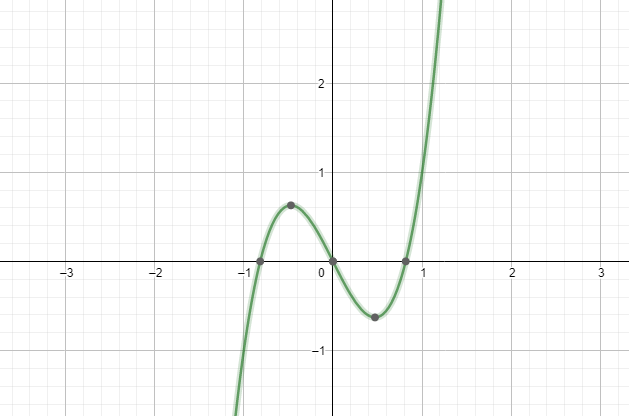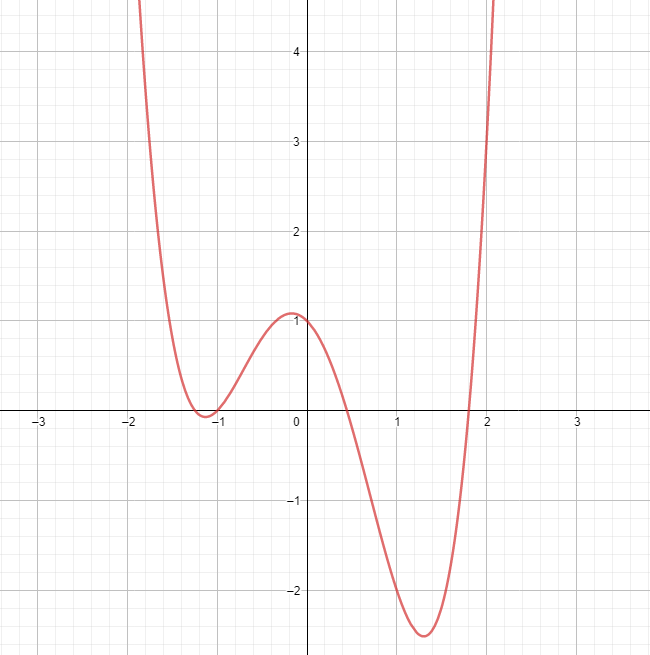• A discontinuous function is a function that has one or more points of discontinuity within its domain.
• Examine the following examples of functions based on their graphs: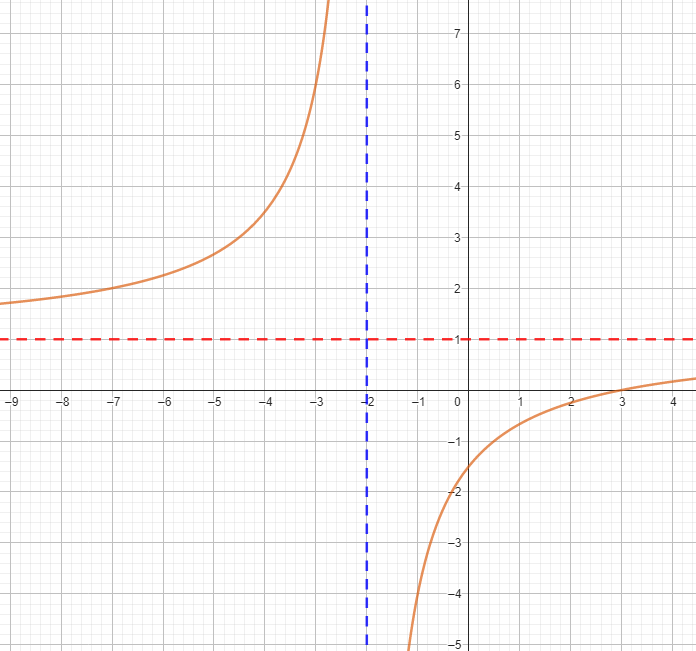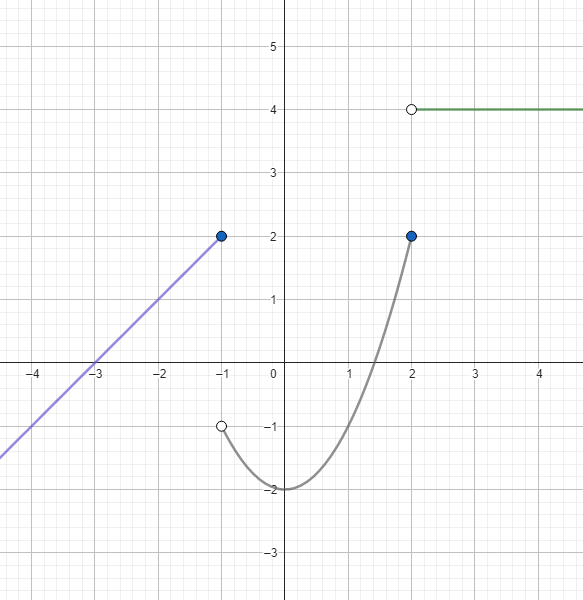## Types of Discontinuity

• In general, there are two types of discontinuities - Irremovable Discontinuity and Removable Discontinuity. Irremovable discontinuities include Jump Discontinuity, Infinite Discontinuity, and Generic Essential Discontinuity). Removable Discontinuity is also called Hole Discontinuity.
• Removable Discontinuity describes a point in the graph of a function that is not defined or does not suit the rest of the graph. This makes a gap in the graph in such a portion of the curve. The example of removable discontinuity is depicted in the graph below.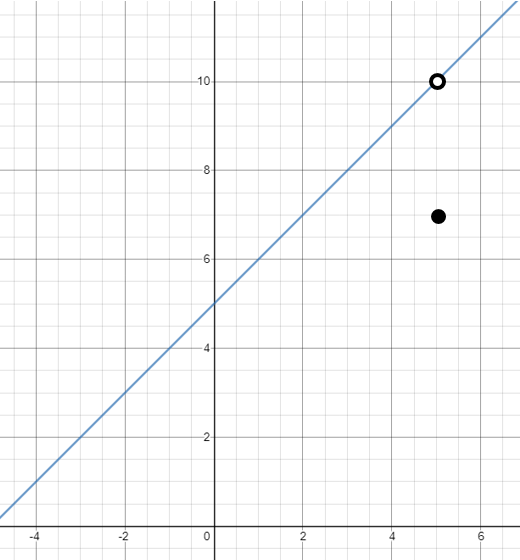• Jump Discontinuity is a type of discontinuity that shows a function that jumps to another location in the coordinate grid. The example of jump discontinuity is depicted in the graph below.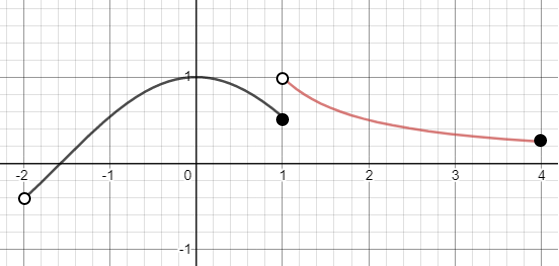• Infinite Discontinuity is a type of discontinuity wherein a function is observed to approach a value that makes the function values increase or decrease indefinitely. Calculus lessons describe one or both one-sided limit approaches to infinity. The example of infinite discontinuity is shown below.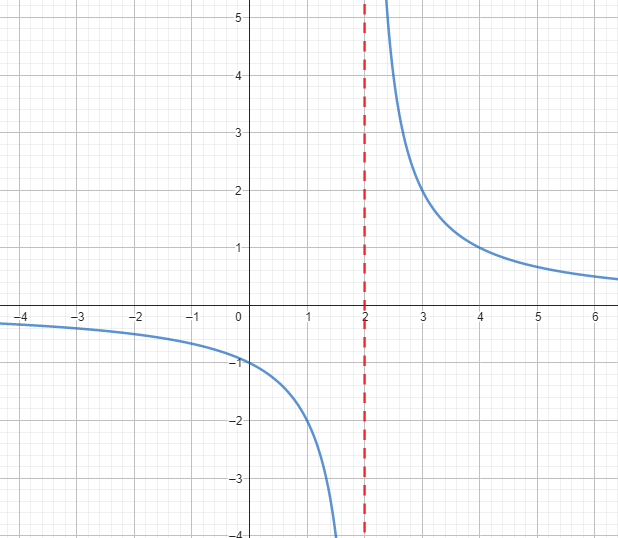• Oscillating Discontinuity is a type of discontinuity that occurs when a function has y-values that approach two or more values simultaneously. Consider the case of $f\left(x\right)=\mathrm{sin}\left(\frac{1}{x}\right)$ below.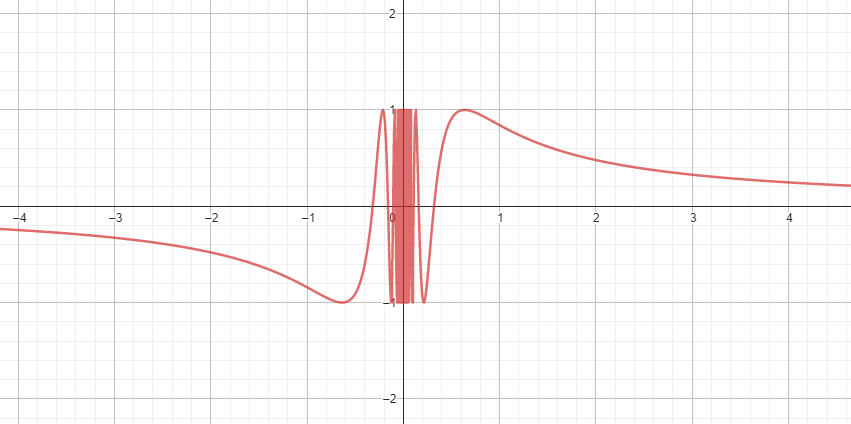## Solved Problems

Example 1. What are the points of discontinuity of the function $f\left(x\right)=\frac{{x}^{2}-7x+6}{{x}^{2}+3x-4}$?

Solution:

$f\left(x\right)=\frac{{x}^{2}-7x+6}{{x}^{2}+3x-4}\to f\left(x\right)=\frac{\left(x-6\right)\left(x-1\right)}{\left(x-1\right)\left(x+4\right)}$

$⇒f\left(x\right)=\frac{x-6}{x+4}$

The canceled factor $\left(x-1\right)$ shows that the function is discontinuous at $x=1.$

The vertical asymptote of $x=-4$ shows that the function is discontinuous at $x=-4$.

The graph is shown below: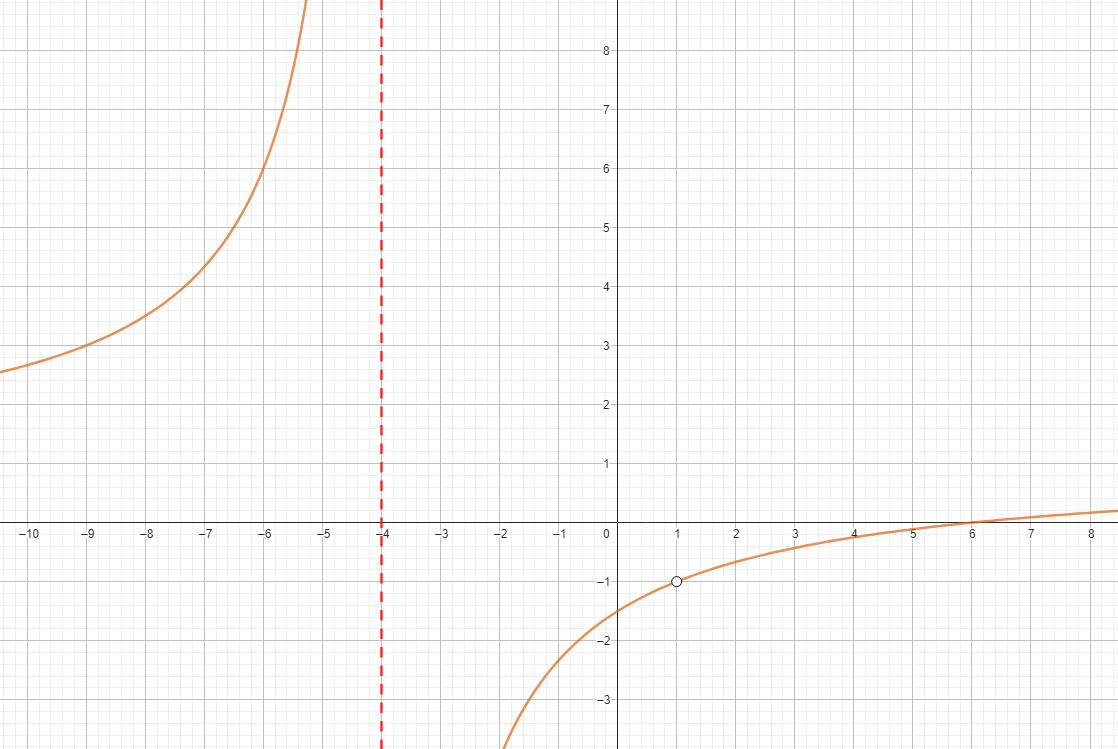The graph shows that the function has an infinite and removable discontinuity.

Example 2. Determine whether the function  is continuous at $x=3.$

Solution:

Substitute $x=3$ to both functions.

$f\left(3\right)={\left(3\right)}^{2}-2=7$

$f\left(3\right)=4\left(3\right)-5=7$

Since both values are equal, then the function is continuous at $x=3.$

## Cheat Sheet

• Rational functions that have restrictions in their domain are discontinuous at those restricted values.
• Polynomial functions are generally continuous at every real number within their domain.
• The example of rational functions like $f\left(x\right)=\frac{\left(x+3\right)\left(x-2\right)}{\left(x+5\right)\left(x+3\right)}$ have both infinite discontinuity and removable discontinuity.
• Square root functions are discontinuous at intervals of real number values that make the radicand negative.
• Cube root functions are continuous at every real number within their domain.
• The example of a function is not continuous for real numbers $x<-2$.
• The cosine and sine functions are continuous, while the tangent and cotangent functions are discontinuous.

## Blunder Areas

• Not all rational functions have points of discontinuity.
• Removable discontinuity and jump discontinuity can be observed in one function.
• Some piecewise functions are continuous, while some are discontinuous.
• Floor functions (Greatest Integer Functions) are not continuous at any integer.
• The continuity of functions is comprehensively discussed in the field of Differential Calculus.
• Limit of Functions is the basic concept required to understand continuous and discontinuous functions in-depth.